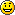## Pairs, sums, and reactivity

I’ve been noodling over a difference between behaviors (functions of time) and events (sequences of time/value pairs). A product of behaviors is isomorphic to a behavior of products, but a product of events is isomorphic to an event of sums.

Ba × Bb ≅ Ba × b

Ea × Eb ≅ Ea + b

A similar property has been noted for Fudgets-like stream processors.

Behaviors (or "reactive values") and events are inter-related. In particular, we can make a behavior from an initial value and an event, using the `stepper` function. If we want to use `stepper` with a pair value, we’d have to use a pair-valued event. However, it’s often more convenient and efficient to work with pair of separate change events, or (using the event pair/sum isomorphism) a sum-valued event.

This post plays with another perspective on sum types for events and stream processors. It can also apply to bots.

### Pairs and sums

Let’s take a look at the event pair/sum isomorphism. To mix two events into a single sum event, simply left- or right-tag the events and merge them:

``mixEither ∷ (Event c, Event d) → Event (Either c d)mixEither (ec,ed) = liftM Left ec `mplus` liftM Right ed``

In this definition, I could have used `fmap` in place of `liftM` and/or `(⊕)` in place of `mplus`. We'll see in a moment that `liftM` and `mplus` give a nicer symmetry.

To separate a sum event into two, use `joinMaybes`.

``joinMaybes ∷ MonadPlus m ⇒ m (Maybe a) → m a``
``unmixEither ∷ Event (Either c d) → (Event c, Event d)unmixEither ecd = (filt left, filt right) where   filt f = joinMaybes (liftM f ecd)``

The functions `left` and `right` are `Maybe`-valued extractors for sum types.

``left  ∷ Either c d → Maybe cright ∷ Either c d → Maybe d``

The mixer and unmixer actually have much more general types:

``mixEither   ∷ MonadPlus m ⇒ (m a, m b) → m (Either a b)``
``unmixEither ∷ MonadPlus m ⇒ m (Either c d) → (m c, m d)``

The simplicity and symmetry these typings motivated my choice of `liftM` and `mplus` above.

In this more general setting, `mixEither ∘ unmixEither` might not be the identity. For lists, all of the lefts will end up before all of the rights. Even for events, `mixEither ∘ unmixEither` can re-order simultaneous occurrences. I don't know how to get a genuine isomorphism.

### Pair editors

When using `stepper`, the event argument indicates a change to the varying value. When the varying value is a pair, we'll often have change events for each pair member. Let's see how to weave those two events into a single pair-valued event.

The pair/sum isomorphism above says that a pair of events is equivalent to a sum-valued event. But what do we do with the sum event? The key idea is noted in Functional reactive partner dancing: convert the sum into a pair editor:

``updPair ∷ Either c d → (c,d) → (c,d)``
``updPair (Left  c') (_,d) = (c',d)updPair (Right d') (c,_) = (c,d')``

Equivalently,

``updPair = (first∘const) `either` (second∘const)``

Now we can go from a pair of events to an event of pair editors:

``pairEditE ∷ (Event c, Event d) → Event ((c,d) → (c,d))pairEditE = liftM updPair ∘ mixEither``

If we want, we can also short-cut the whole sum business:

``pairEditE (ce,de) =  liftM (first∘const) ce `mplus` liftM (second∘const) de``

With either of these definitions, we have a much more general type:

``pairEditE ∷ MonadPlus m ⇒ (m c,m d) → m ((c,d) → (c,d))``

The next step is to apply successive functions from the pair-changing event, using an accumulation combinator. We'll need an initial value for the pair:

``pairE ∷ (c,d) → (Event c, Event d) → Event (c,d)cd `pairE` cde = cd `accumE` pairEditE cde``

There's another way to dice up `pairE`. A reactive value is determined by an initial value and change event, so we can restructure the arguments of `pairE` into two reactive values. We also have an initial value for the combination, so we may as well produce a reactive value.

``pairR ∷ Reactive c → Reactive d → Reactive (c,d)(c `Stepper` ce) `pairR` (d `Stepper` de) =  (c,d) `stepper` pairE (c,d) (ce,de)``

or, more directly:

``(c `Stepper` ce) `pairR` (d `Stepper` de) =  (c,d) `accumR` pairEditE (ce,de)``

Lovely!

### Pair editing and applicative functors

In a sense, we've gone to a lot of work for nothing. Since `Reactive` is an applicative functor, we can also give this trivially easy and much more general definition:

``pairR ∷ Applicative f ⇒ f c → f d → f (c,d)pairR = liftA2 (,)``

On the other hand, the `(<*>)` method in the `Applicative` instance given in Reactive normal form was tricky. We can now replace it with a simple definition in terms of `pairR` (assuming we don't define `pairR` in terms of `liftA2`). Use `pairR` to turn a reactive function and a reactive argument into a reactive function/argument pair. Then map uncurried function application to get `(<*>)`:

``rf <*> rx = uncurry (\$) <\$> (rf `pairR` rx)``

I like how these new definitions of `pairR` and `(<*>)` build on other generally useful combinators. In contrast, my previous `(<*>)` definition is recursive and uses the representation of reactive values and events.

(c,d) `accumR` pairEditE ( ce ,de)
still, very nice catch – thank you for the write-up!